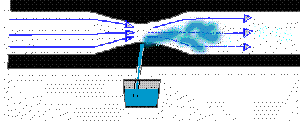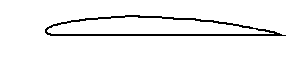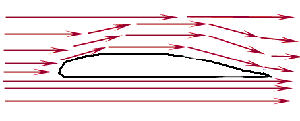lift

Meteo

Support is based on some important factors: the wing profile, the angle of attack and airspeed.
The law of support is based on the Bernoulli's theorem and flow Equation. Bernoulli's theorem says: “The higher the air flow rate, the greater the dynamic pressure will be lower and the static pressure”. The flow of the equation says that the narrower flow pipe, the greater the velocity of the fluid and vice versa.
For explanation purposes:
• the dynamic pressure that is produced by moving air. When hitting any object, this air will produce a certain pressure. This is the dynamic pressure.
Pd=1/2D x V²
where Pd = dynamic pressure ; D = air density at flight level ;
V = velocity relative to the air;
• static pressure that is produced by the concentration of air molecules. this pressure, for use in aviation, It is the atmospheric pressure.
This theorem can be proved in practice through the venturi. It consists of a pipe with a narrowing in the middle, where we make a small hole, adapting a plastic straw dipped in a glass of water.Note that the air accelerates the narrowing (most dynamic pressure), causing a suction pipe in (reduction in static pressure), which in turn sprays water into the tube.
Now that we know that the air flows faster into narrower surfaces, we can begin to understand what makes a paraglider flying. Let's look at the profile of the wing of an airplane:wings profiles can be in two ways: The profiles of the two equal sides are called symmetric, and profiles with different format as the sides of the figure, They are called asymmetric.
The elements of a profile of a wing are:
• Board of Attack: And the front end of the profile, where the air hits first.
• Board of Fuga: And the rear end of the profile, through which air flows.
• Extradorso: It is the top surface of the profile, the top side.
• Intradorso: It is the lower surface of the profile, the bottom side.
• Rope: It is an imaginary straight line connecting the leading edge to the trailing edge.
• Mean Curvature Line: It is also the line that separates the upper surface of the soffit.
To understand support, We suppose that the air hit at a certain speed on a profile, which in this example is asymmetrical. Upon reaching the leading edge, air flows to the extrados and intrados. Note that the path to be traveled by air in the lower surface is less than the upper surface, at where, because the curvature of the wing, the path will be higher.
We determine it to be enough that we took a tape measure and measure the rope in an asymmetric wing upper surface and the lower surface. Let's say hypothetically that the measurement was the soffit 1,24 m. I do not extradorso, the measurement would 1,33 m.
As we saw no Venturi tube, the flow tube estreitarmos, the air accelerates. As the kite will be wrapped in air, the upper layer to that flowing over the surface will act as the walls of the venturi. Observe Figure:air, enclosed between the layers of air just above, accelerates not extradorso, while the straight intrados surface suffers a minimum acceleration of the flow. Recalling again the theorem of Bernoulli, that the greater the dynamic pressure, the lower the static pressure, the result will be the static pressure in the upper surface lower than the static pressure in the soffit.
As static pressure acts on all sides in all directions, the upper surface it will act from top to bottom, soffit and not act from the bottom up. Because the static pressure in the soffit (upwards) is greater than the static pressure at the extrados, the wing will win support. This lift force must be equal to the weight of the set to make it fly straight.